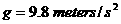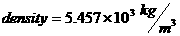# Calculate the density of Earth from Newton’s law of gravitation

Dear Student
Assuming earth to be a perfect sphere of radius R and uniform density p,
mass of Earth=volume of Earth x density,• The average radius of the Earth is 6.38 x 106 meters

• The universal gravitational constant G is Nm2/s2
• The acceleration of gravity at the surface of the Earth is aboutSubstitute the values we will getRegards

• 1